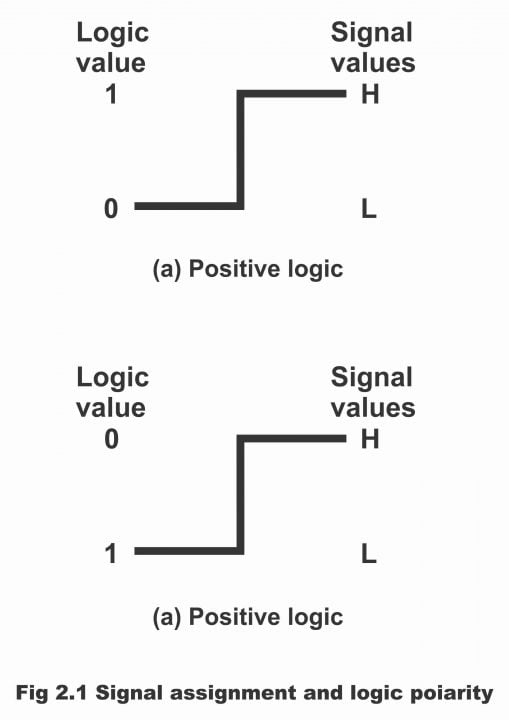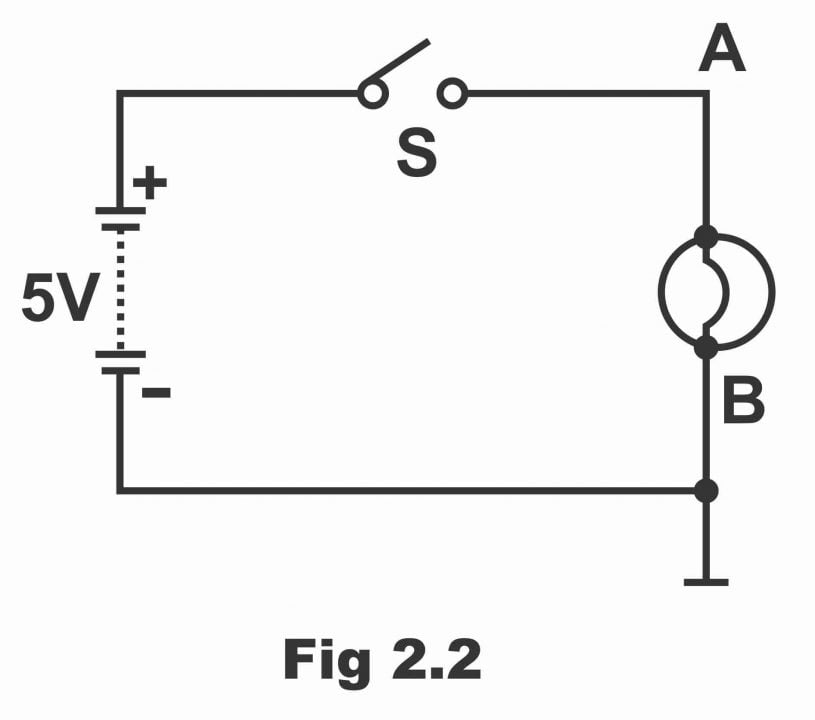Digital Electronics

# Introduction to Logic Gate with Examples

## Definition of a Logic Gate

A logic gate is a kind of electronic circuit, which makes logical decisions. In simple words, an electronic switching circuit, which is provided digital inputs (i.e. having two states 0 or 1), is called a logic gate. (Remember that the term logic is usually used for certain decision-making processes, whereas the term gate is used for such a circuit, which performs the basic logic operation). As such, a logic gate is a circuit, which decides whether an output should be treated as Yes or No on the basis of its provided inputs. The logic gate just provides one output according to the number of inputs whether one or more inputs have been provided on its input side (i.e. it has just one output and has one or more than one input). In other words, output received from a logic gate is a combined outcome of one or more than one input having been applied.

A logic gate is an electronic circuit that makes logical decisions. In other words, a logic gate is an electronic circuit, having one or more inputs and only one output, which can either be high or low (based on the input status). Logic gates are widely used in digital computers and in different types of digital systems and circuits.

Logic gates are basic building blocks, on the basis of or with the help of which, digital systems are designed. Logic gates carry out hardware logic functions through the application of logical algebra or Boolean algebra (remember that Boolean algebra was introduced by George Boole in 1854, that’s the reason it is known as Boolean algebra) which helps computers a great deal in decision-making and perform required computing operations in a short period of time. In other words, the entire hardware logic function depends on logic algebra and the logic gates carry out the hardware logic function in a jiffy on the basis of this algebra. An extraordinary characteristic of Boolean algebra is that it has just two values of variables being used in it (either 0 or 1). Therefore, every variable is either zero or one (i.e. the value of each variable is either zero or it is 1). In other words, two binary digits are used in Boolean algebra in order to reflect the two levels having taken place on a digital circuit, out of which binary 1 represents high level whereas binary 0 denotes low level.

Nowadays, these gates are available in a variety of IC families (that’s these gates belong or are related to different IC families), the most popular or famous of which are the following families.

1. Transistor- Transistor Logic or TTL
2. Emitter Coupled Logic or ECL
3. Metal Oxide Semiconductor MOS
4. Complementary Metal Oxide Semiconductor CMOS

The detail of the aforementioned IC families will be presented in the coming articles

## Positive and Negative Logic

The digits 0 and 1 have paramount standing in computing systems, which represents two possible states of a device or circuit. If these two states are represented as ON and OFF, Closed and Open, High and Low, Plus and Minus, True and False or Up and Down, even then no difference occurs. However, it is a settled point that these two states are represented by two distinct words or two distinct digits. Thus, 1- symbol in a positive logic may reflect the following:

i). An ON circuit

ii). A closed switch

iii). High voltage

iv). A plus sign

v). a true statement

vi). Up

On the contrary, the 0-symbol in positive logic represents the following:

i). An off circuit

ii). Open switch

iii). Low voltage

iv). A minus sign

v). A false statement

vi). Down

Remember that a completely reversed condition takes place in case of negative logic.

Thus, positive and negative logic can be defined as follows:

If in any digital or computing system, off circuit or open switch or low voltage is represented by binary 0 and an on the circuit, closed switch or high voltage by binary 1, it is called positive logic.

On the contrary, if in any digital circuit or computing system, off circuit or open switch or low voltage is represented by binary 1 and on circuit or closed switch or high voltage by binary 0, it is called negative logic. This has been exemplified in figure 2.1

Figure 2.1 – signal assignment and logic polaritySuppose that a digital system consists on two voltages level 0V and 5V. If symbol 1 is reserved for 5V and symbol 0 is considered as 0V, in such a situation it becomes a positive logic system. On the contrary, if we set symbol-1 as 0V and symbol-0 as 5V, in such a situation we will have a negative logic system. This point can also be expounded with the help of a simple electrical circuit shown in figure 2.2. In this circuit, a lamp has been fitted between terminal A and B through a 5V battery and a switch S. terminal B has been earthed. When the switch opens, 0 volts are received on terminal A with respect to earth, thus lamp does not illuminate. However, when the switch closes, +5V are received on terminal A with respect to its earth and the lamp starts to irradiate. As such, two likely output states (i.e. oV or +5V) emerge with respect to earth or terminal B.

Now assume that the battery displayed in the diagram has been reversed. In such a situation, two possible output states (i.e. 0V and -5V) result. Again, we represent symbol-0 by 0V and symbol-1 by -5V.

Figure 2.2It should be kept in mind that whichever of the two voltage levels is more positive in positive logic, it denotes 1. Whereas in the situation of negative logic, the voltage level, which is more negative, denotes 1. Moreover, it is also not necessary that symbol – 0 be represented by 0V every time, rather symbol – 0 and symbol -1 can sometimes be presented by some other digits as well. For example, suppose that two voltage levels 2V and 10V exist in a circuit. In this case, symbol -1 reflects high voltage (10V) and symbol – 0 low voltage (2V). if the values of voltage levels are -2V and -8V, then in the case of positive logic, symbol – 1 reflects -2V whereas symbol – 0 -8V (i.e. the lowest of the two voltage values). It has to be remembered that negative logic seems somewhat unnatural, (though it has multiple applications), therefore here we primarily concentrate on positive logic for the sake of simplicity.

Previous Topic: Basic Elements of Digital Electronics

Next Topic: Logic AND Gate Working Principle & Circuit Diagram

For electronics and programming-related projects visit my YouTube channel.